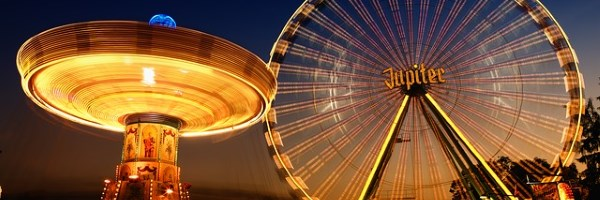# Picture StoryTake a look at the video below.

Can you describe what happens?

Can you explain how the video might continue, if more little cubes were available?

Can you be sure that the patterns in the video will carry on?

Can you use algebra to represent the patterns you saw?

1 cubed has 1 cube in it which is also a square number. 2 cubed has 8 cubes in it, 8 + 1 = 9 which is also a square number.
3 cubed has 27 cubes in it, 27 + 8 + 1 = 36 which is also a square number...So, 6 cubed has 216 cubes in it, 216 + 125 + 64 + 27 + 8 + 1 = 441 which is also a square number. The pattern will continue forever as long as the cube keeps increasing by one.### Can you be sure?

Can you be sure that the answer will always be a square number? How do you know?

### Yes

1^3+2^3+3^3+...+n^3 = (1+2+3+...+n)^2

Can you describe what happens?
The pattern starts as 1 cubed(1), then 2 cubed(8) is added onto the 1. This equals 9. Next, 3 cubed is added on to the 9. This pattern happens again and again.

Can you explain how the video might continue, if more little cubes were available?
The overall square would get bigger and bigger.

Can you be sure that the patterns in the video will carry on?
Yes.Because of the algebra I will show you in a sec.

Can you use algebra to represent the patterns you saw?
(Total of little squares in a overall square)=n*n*n+(n-1)*(n-1)*(n-1)+(n-2)*(n-2)*(n-2)+(n-3)*(n-3)*(n-3)*...### How big is the square?

Can you describe how the overall square's size relates to n?

### Can you explain what happens?

The cubes go up by 1 number cubed each time.

First it is 1 cubed and then 2 cubed. The result of adding these numbers equals 9, which is 3 squared.

Then by adding 3 cubed you end up with 27 more cubes. That's 36 altogether, which is 6 squared.

And this cycle continues.

### Can you explain what happens?

Can you explain why the cycle will continue?
How can you be sure that the little cubes will always add up to a square number?